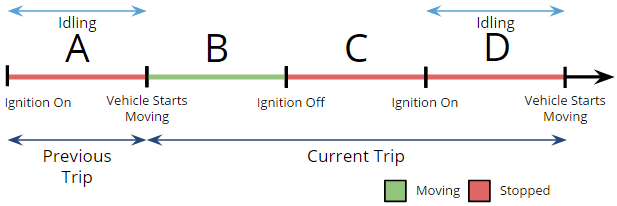# Geotab - How does the system calculate idling time?

Idling occurs when the engine is running, but the vehicle is not changing position. Idling that occurs before a trip starts is included as part of the idling for the previous trip. Please review the examples below to help understand how idling time gets attributed to particular trips.

Examples

#### Example — Time Spent Idling Before and After DrivingIn this example, let’s assume time A and D are times spent idling. Therefore:revious trip idle time = A

Current trip = B + C + D

Current trip idle time = D

#### Example — Time Spent Idling in One TripIn this example, let’s assume time B and D are times spent idling. Therefore:

Current trip = A + B + C + D

Current trip idle time = B + D

#### Example — Time Spent Idling During Two TripsIn this example, A+B is the first trip, and C+D is the second trip. The idle times are B and D where B is attributed to the first trip, and D is attributed to the second trip.

Did you find it helpful? Yes No

Send feedback